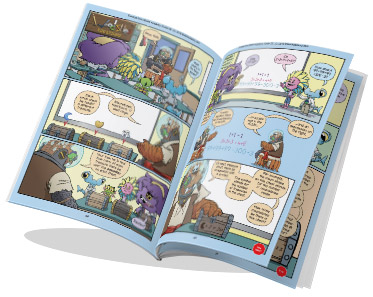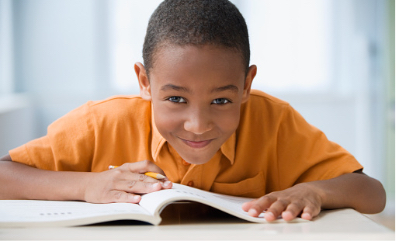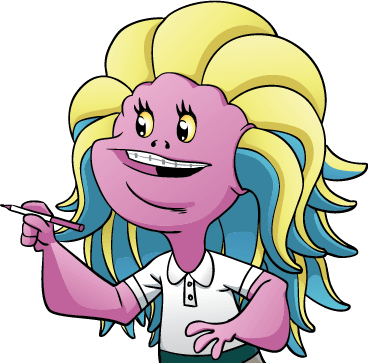## Level 2 Books Information

Designed in an engaging comic-book style format, our Level 2 curriculum includes the Guide books and Practice books for all four units (2A, 2B, 2C, 2D).

• The Guide book is a full-color paperback textbook, which teaches concepts in a comic-book style.
• The Practice book complements the Guide book and includes 300+ problems ranging from introductory-level exercises to challenging puzzles and word problems.Level 2 has four units: A, B, C and D. Topics build upon each other from unit to unit.

2A
2B
2C
2D
Place Value
Digits, ones, tens, hundreds, breaking and regrouping, adding and subtracting 1, 10, or 100.
Subtraction
Place value strategies, using addition, finding a difference, counting up, subtracting then adding, subtracting all at once, and subtracting in parts.
Measurement
The measurement chapter only includes measuring length, and includes using a ruler, estimation, customary and metric units, conversions, mixed measures, and adding and subtracting.
Big Numbers
Thousands, millions and larger place values. Reading, writing, adding, subtracting, comparing, and estimating with big numbers.
Comparing
The number line, distance, consecutive numbers, using < and >, ordering numbers.
Expressions
Evaluating expressions, using parentheses, working with symbols, simplifying, and solving equations.
Strategies
Rearranging addition and subtraction, canceling, almost canceling, subtracting everything at once, using parentheses, and skip-counting.
Algorithms
Stacking addition with regrouping, adding more than two numbers at a time, stacking subtraction with breaking, and solving cryptarithms.
Sums, place value strategies, counting up, adding and taking away, doubles, making 10's and 100's, friendly sums.
Problem Solving
Guessing and checking, working backwards, drawing a picture, finding useful information.
Odds & Evens
Odds and evens, doubling, halving, addition and subtraction patterns, working with more than two numbers, and puzzles involving crossings, coins, checkerboards, and graphs.
Problem Solving
Staying organized, finding and using patterns, solving simpler problems, eliminating choices, and using manipulatives.

We recommend taking the Level 2A Placement Test to determine if your student is ready to take Beast Academy Level 2.

Solutions to the placement test are found at the end of the test. Your student may also take the Level 2B, 2C, or 2D placement tests to determine readiness per unit.Get the guide books for sure, because they are endearing and interesting and adorable and wonderful and they make your kid fall in love with doing math (even if they already love math).

Kjersti, BA parent